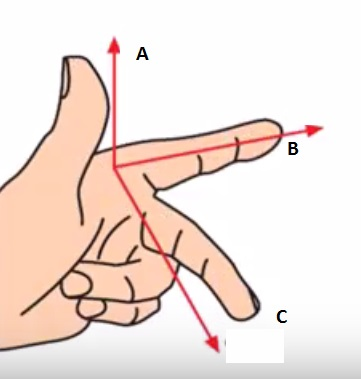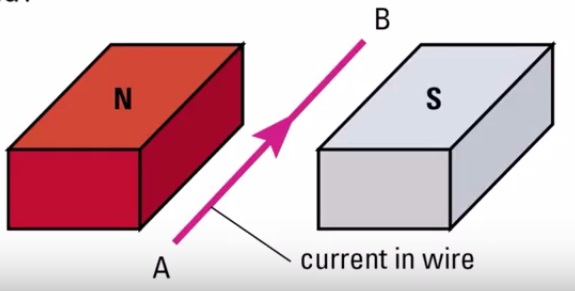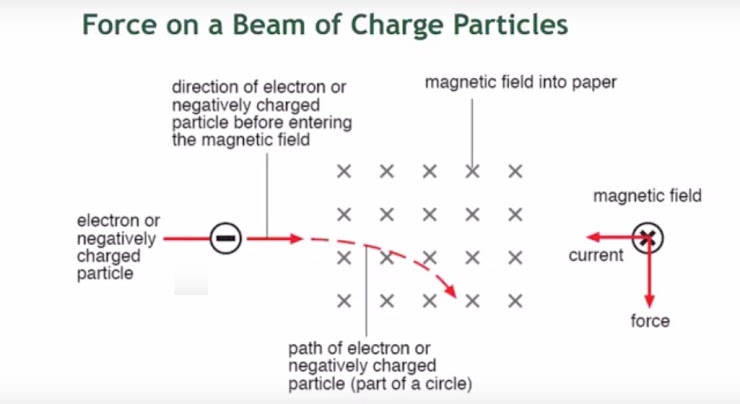Magnetism and Electromagnetism Part 11
The force acting on a current carrying conductor in a magnetic field is *
1 point
The direction of the force is reversed *
1 point
Circuit Breakers are designed for *
1 point
What does A represent? *
1 pointWhat does C represent? *
1 pointWhat is the direction of the force exerted? *
1 pointWhat is the direction of the force exerted if the current is reversed from B to A? *
1 pointThe diagram shows a force on an electron beam. Why is the force acting downwards using Fleming's Left hand Rule? *
0 points